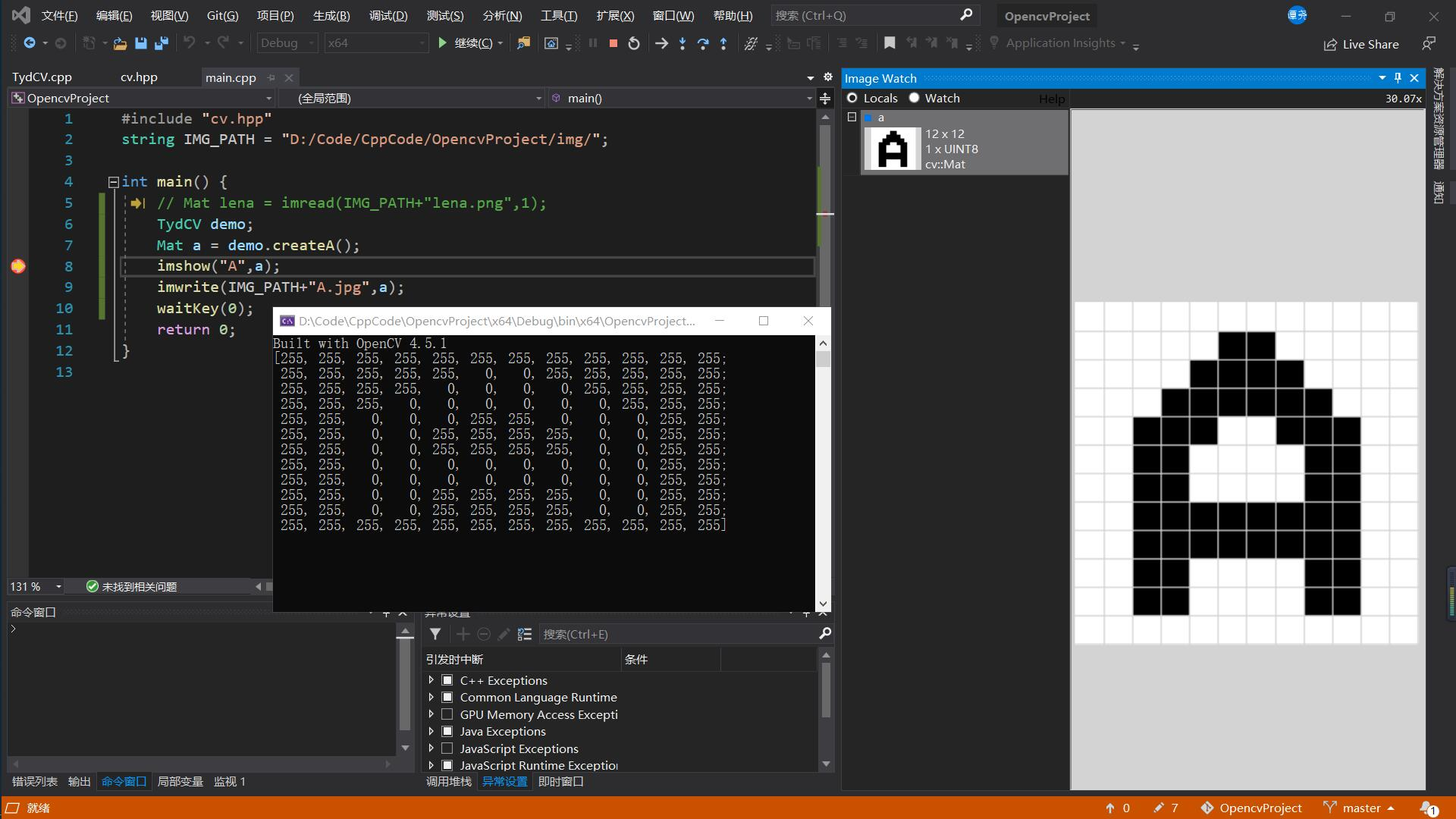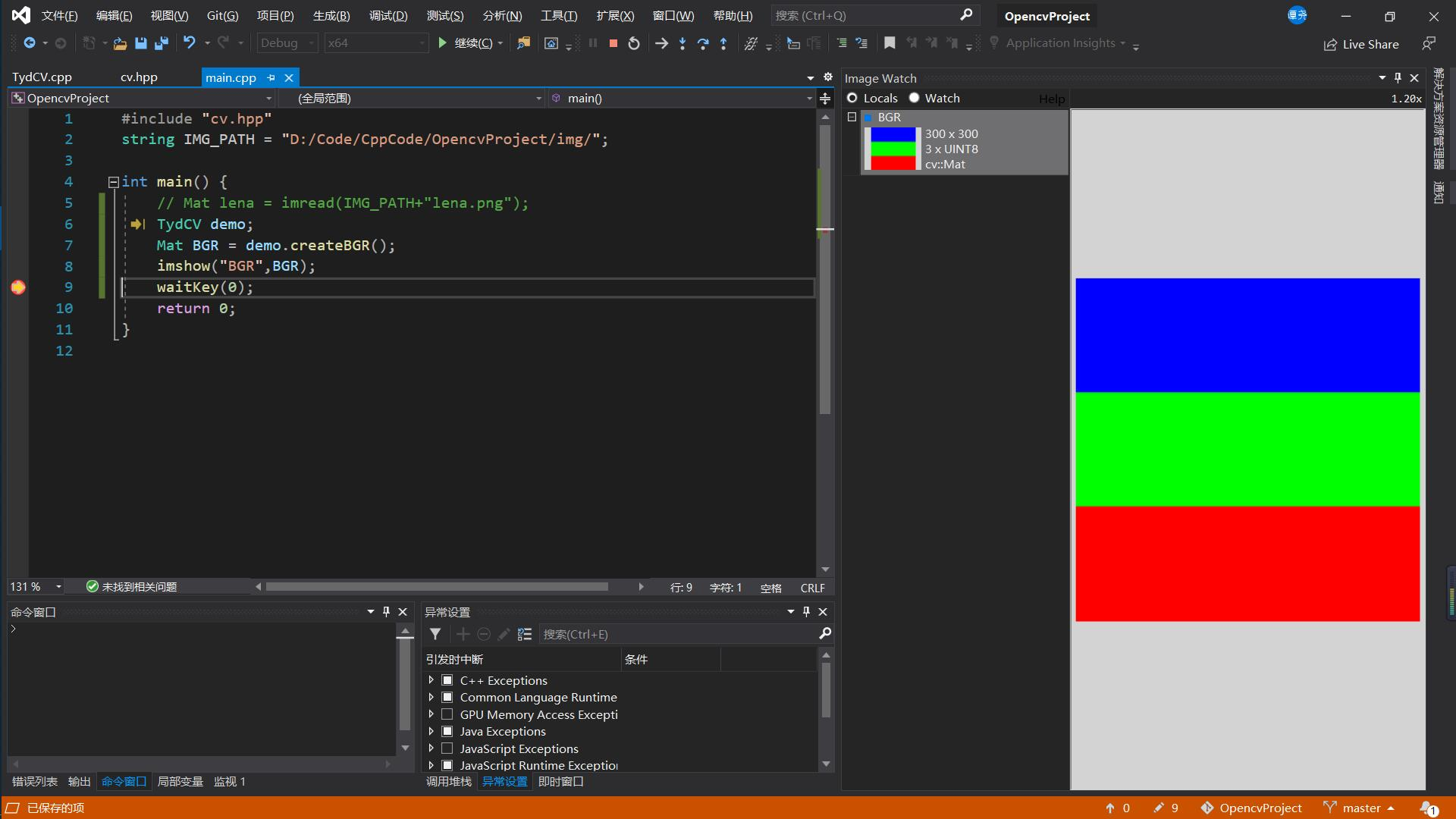### 前言

OpenCV 本身是一个【黑盒】，它为我们提供了图像处理的接口（参数、返回值）。我们只需要掌握接口的正确使用方法，就可以在完全不了解其内部工作原理（算法）的情况下，方便地进行各种复杂的图像处理。但是如果我们完全忽略内部工作原理是如何实现的，是不可能很好地使用OpenCV的，更不可能设计出好的计算机视觉应用系统。因此从上述角度讲，我们可以从两个角度学习 OpenCV：

● 将 OpenCV 作为【白盒】学习：深入学习 OpenCV 每个函数所使用算法的基本原理、每个函数的具体实现细节，进一步加深对图像处理的理解。
● 将 OpenCV 作为【黑盒】学习：仅仅将 OpenCV 作为一个工具来使用，学习的是每个函数内参数的含义和使用方式，学习的目的是更好地使用 OpenCV 函数。

### 二值图像

``````Mat a = Mat(12, 12, CV_8UC1, 255);   //创建空白灰度图像
``````### 彩色图像

● 第 1 个通道是 B 通道
● 第 2 个通道是 G 通道
● 第 3 个通道是 R 通道

``````//这是一段没有任何作用的代码，只是用来了解这个层次
Mat image = Mat::zeros(12, 12, CV_8UC3);  //3通道的RGB图像
int width = a.cols;  //列数，反映矩阵宽度
int height = a.rows; //行数，反映矩阵高度
int dims = a.channels();
cout << "width:" << a.cols << ",height:" << a.rows << ",channels:" << a.channels() << endl;
for(int row = 0; row < width; row++) {
for(int col = 0; col < height; col++) {
Vec3b bgr = image.at<Vec3b>(row,col);
int B = image.at<Vec3b>(row,col);   //B通道数据
int G = image.at<Vec3b>(row,col);   //G通道数据
int R = image.at<Vec3b>(row,col);   //R通道数据
}
}
``````

``````Mat TydCV::createBGR() {
Mat a = Mat(300, 300, CV_8UC3, Scalar(0,0,0));
int width = a.cols;
int height = a.rows;
for(int row = 0; row < width; row++) {
if(row < 100) { //0<=row<100
for(int col = 0; col < height; col++) {
Vec3b bgr = a.at<Vec3b>(row,col);
a.at<Vec3b>(row,col) = 255;
}
} else if(row < 200) { //100<=row<200
for(int col = 0; col < height; col++) {
Vec3b rgb = a.at<Vec3b>(row,col);
a.at<Vec3b>(row,col) = 255;
}
} else { //200<=row<300
for(int col = 0; col < height; col++) {
Vec3b rgb = a.at<Vec3b>(row,col);
a.at<Vec3b>(row,col) = 255;
}
}
}
return a;
}
``````